# SSAT Elementary Level Math : How to find the whole from the part

## Example Questions

### Example Question #8 : Compare Two Objects With A Measurable Attribute: Ccss.Math.Content.K.Md.A.2

Which shape is bigger?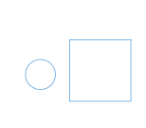They are the same size

The circle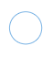The square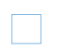The squareExplanation:

The square is bigger than the circle.

### Example Question #9 : Compare Two Objects With A Measurable Attribute: Ccss.Math.Content.K.Md.A.2

Which shape is bigger?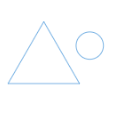The circleThey are the same size

The triangle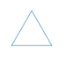The triangleExplanation:

The triangle is bigger than the circle.

### Example Question #10 : Compare Two Objects With A Measurable Attribute: Ccss.Math.Content.K.Md.A.2

Which shape is bigger?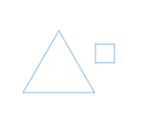The squareThey are the same size

The triangleThe triangleExplanation:

The triangle is bigger than the square.

### Example Question #61 : Whole And Part

Which line is longer?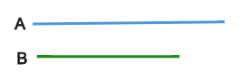They are the same length

Line A

Line B

Line A

Explanation:

Line A in longer than line B.

### Example Question #21 : Describing And Comparing Measurable Attributes

Which line is longer?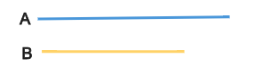Line A

They are the same length

Line B

Line A

Explanation:

Line A is longer than line B.

### Example Question #63 : Whole And Part

Which line is longer?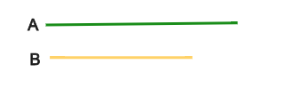Line A

They are the same length

Line B

Line A

Explanation:

Line A is longer than line B.

### Example Question #64 : Whole And Part

Which line is longer?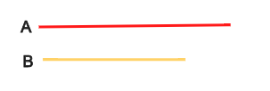Line A

They are the same length

Line B

Line A

Explanation:

Line A is longer than line B.

### Example Question #65 : Whole And Part

Which line is longer?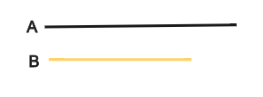Line B

They are the same length

Line A

Line A

Explanation:

Line A is longer than line B.

### Example Question #66 : Whole And Part

Which line is longer?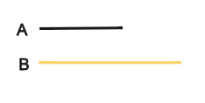They are the same length

Line A

Line B

Line B

Explanation:

Line B is longer than line A.

### Example Question #67 : Whole And Part

Which line is longer?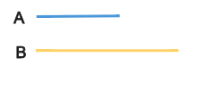Line B

Line A

They are the same length